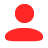## 一、有限元能干什么

；

；

，称之为狄利克雷边界条件；一类是变量的导数有约束，如

，称之为诺依曼边界条件；还有第三类就是前两者的混合，成为罗宾边界条件。

## 二、偏微分方程如何求解（有限元发明以前）

主要用来模拟非边界条件的点，它们在边界处取值应该为零，否则和

叠加后会破坏位移边界条件。

为待定系数。

组合就会获得不同的位移，这样我们就可以列出总势能的表达式，其中只包含待定系数

，由前面我们说的最小势能原理，

只有一组是真实的解，那就是当总势能变分

求导取零就行了，这个时候偏微分方程组就变成线性方程组了，这种解偏微分的方法称之为利兹法。

；

，得到

## 三、有限元法的基本思路

，所以当

。也就是说我们可以采用“化整为零”的思想，将复杂不易求解的东西切成简单的容易计算的几何形状来进行等效。

，试函数的要求也不高（连续性阶次较低），缺点就是计算量变大了，不过我们现在有计算机了，这都不是事。

 曾攀-有限元分析基础教程

## 四、有限元法的数学基础——降维

，它的特点是在节点

)处取0，其他位置线性变化，形状曲线如下：

Source：lectures of Prof. Mats G. Larson

，于是可以得到：

Source：lectures of Prof. Mats G. Larson

，

，

，

,同样可以计算

MATLAB代码如下：

``````function M = MassMat1D(x)
n = length(x)-1; % number of subintervals
M = zeros(n+1,n+1); % allocate mass matrix
for i = 1:n % loop over subintervals
h = x(i+1) - x(i); % interval length
M(i,i) = M(i,i) + h/3; % add h/3 to M(i,i)
M(i,i+1) = M(i,i+1) + h/6;
M(i+1,i) = M(i+1,i) + h/6;
M(i+1,i+1) = M(i+1,i+1) + h/3;
end``````

MATLAB代码如下：

``````function b = LoadVec1D(x,f)
n = length(x)-1;
b = zeros(n+1,1);
for i = 1:n
h = x(i+1) - x(i);
b(i) = b(i) + f(x(i))*h/2;
b(i+1) = b(i+1) + f(x(i+1))*h/2;
end``````

,代码如下：

``````function y = Foo(x)
y=x.*sin(x)``````

``````n = 5 ;% number of subintervals
h = 1/n; % mesh size
x = 0:h:1; % mesh
y = Foo(x);% calc f(x)
M = MassMat1D(x); % assemble mass
Pf = M\b; % solve linear system
plot(x,Pf,x,y); % plot  projection``````

 Larson, Mats G., and Fredrik Bengzon. "The finite element method: theory, implementation, and practice."

## 五、如何获得“弱形式”的解

为给定的函数，

，

，

（任意的），将原方程改写成如下方式：

，然后通过求取试函数的系数

，则很容易得到

，

，则有

，即

MATLAB代码如下：

``````function A = StiffMat1D(x,kappa)
n = length(x)-1;
A = zeros(n+1,n+1);
for i = 1:n
h = x(i+1) - x(i);
A(i,i) = A(i,i) + 1/h;
A(i,i+1) = A(i,i+1) - 1/h;
A(i+1,i) = A(i+1,i) - 1/h;
A(i+1,i+1) = A(i+1,i+1) + 1/h;
end
A(1,1) = A(1,1) + kappa(1);
A(n+1,n+1) = A(n+1,n+1) + kappa(2);``````

MATLAB代码如下：

``````function b = LoadVec1D(x,f,kappa,g)
n = length(x)-1;
b = zeros(n+1,1);
for i = 1:n
h = x(i+1) - x(i);
b(i) = b(i) + f(x(i))*h/2;
b(i+1) = b(i+1) + f(x(i+1))*h/2;
end
b(1) = b(1) + kappa(1)*g(1);
b(n+1) = b(n+1) + kappa(2)*g(2);``````

，这就需要一点数学技巧了！

，那

，所以只要我们设置

``````function y = Source(x)
y = 0.03*(x-6)ˆ4; % heat source``````

``````%%solve Poission equations
h = 0.1; % mesh size
x = 2:h:8; % mesh
kappa = [1.e+6 0];
g = [-1 0];
A = StiffMat1D(x, kappa);
b = LoadVec1D(x, @Source, kappa, g);
U = A\b;
plot(x,U)``````

 Larson, Mats G., and Fredrik Bengzon. "The finite element method: theory, implementation, and practice."

## 六、二维、三维有限元计算

Source：An Introduction to the Finite Element Method（Comsol）

Source：An Introduction to the Finite Element Method（Comsol）

Source：An Introduction to the Finite Element Method（Comsol）zZ  回复 2021-07-25 22:49:12